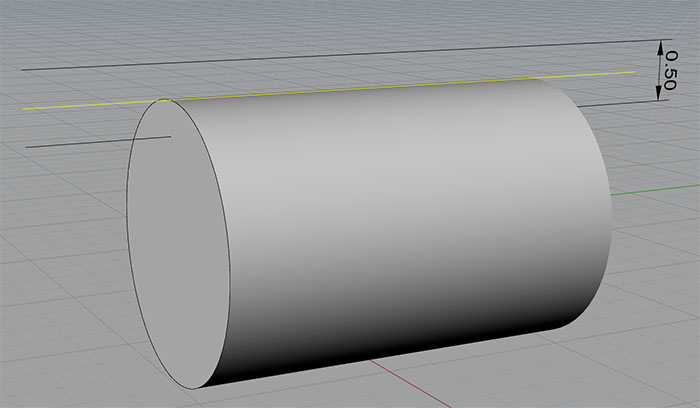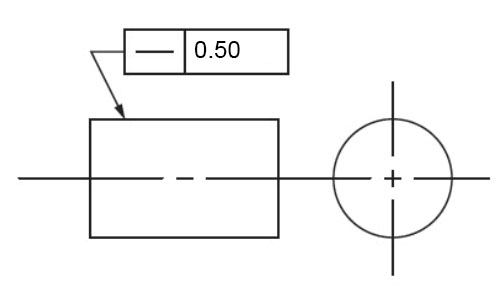# Easy Guide to GD&T – Straightness [ Symbol, Tolerance, Measurement ]

The GD&T Straightness Control is a Form control, meaning it controls the shape of the feature or surface and defines the shape’s allowable deviation from its ideal form.

Straightness is the only callout that can control either lines on a surface or a feature of size (FOS).  In other words, Straightness GD&T can be used for two very different functions–Surface Straightness and Axis Straightness.

## GD&T Surface Straightness

Surface Straightness is a tolerance that controls a line somewhere on a surface or a feature. For example, suppose we are controlling Straightness Tolerance on a cylindrical part or feature of a part. Pictorially, here’s what that means:Straightness Tolerance is the maximum deviation from the yellow line on the cylindrical surface…Surface Straightness callout for the drawing above…

When we specify the surface flatness, the tolerance zone will form a two dimensional wide area above and below the ideal surface position and control all deviations. Surface straightness controls the form of lines anywhere on the surface, and there are two types of applications:

• The first is a flat surface, such as the surface of a cube.
• The second is the axial cylindrical surface. The Surface Straightness callout points the arrow to the surface and gives the tolerance.

In both cases, the tolerance zone forms a 2D plane. It appears as two parallel lines (also parallel to the surface), one above the surface and the other below the surface.

The Surface Straightness callout or feature control frame points the arrow to the surface and gives the tolerance zone size.

## GD&T Axis Straightness

Axis Straightness, sometimes called “axial straightness”, is the tolerance that controls how much curve is allowed in a part’s axis.

Imagine the same sort of drawing as what we have above, only the line is on the axis rather than the surface. Now we are controlling the Axis Straightness. We specify how much it may vary from the ideal, just as with Surface Straightness. One other difference is that the axis may vary in more than one dimension, so the tolerance zone must be cylindrical around the line, rather than just being above and below the line.  Therefore the Feature Control Frame has both the tolerance value, and the diameter symbol to specify properly that the tolerance zone is cylindrical.

Another difference is that the leader will point to the part’s diametric size dimension rather than the axis.

When using Axis Straightness, MMC is often called out.

When called out in this way, the Straightness Tolerance is referred to as Derived Median Line Straightness.

## Gaging and Measurement of GD&T Straightness

Surface Straightness is pretty easy to gage–we can simply sweep an indicator along the Straightness line called out and measure the deviation in that way.  The Tolerance Zone is the two dimensional area centered on the line you would sweep.

Axis Straightness is typically measured with a cylindrical gage made just enough larger than the diameter of the feature to accommodate the variation that’s allowed. By using an MMC callout, we can ensure that both by virtue of straightness and dimensionally, the part will always fit in a given size hole. Therefore, if you make a part smaller in OD, you gain bonus tolerance which allows the part to be correspondingly less straight.  This is typically done with a Go-No Go gage such as what’s described in this video:

The Tolerance Zone is a 3 dimensional cylinder checked via Go-No-Go Gage…

Alternatively, a set of dial gages or a CMM machine can be used.

## Usage Notes

In some sense, Surface Straightness is a 2-dimensional version of Flatness. Both are measured without datums and both are used to control and refine the size of a feature. In the same sort of way, we can consider Axis Straightness to be related to Axis Parallelism and Perpendicularity since these are all concerned with controlling a center axis within a cylindrical tolerance zone.

Surface Straightness is very commonly used for surfaces that have to mate with another part while providing a seal. Think hydraulic sleeves, tubes, and caps.

Axis Straightness, OTOH, is most commonly used when designing pins that must fit in some specified bore.